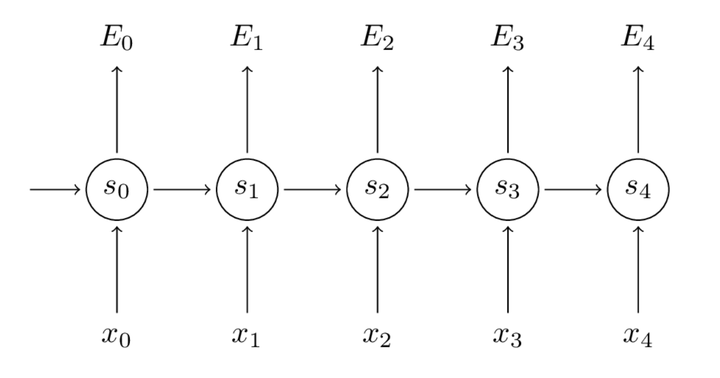# 一文搞懂 RNN（循环神经网络）

## 目录

1 基础篇

• 神经网络基础
• 为什么需要RNN（循环神经网络）
• RNN结构
• 小结

2 RNN的反向求导过程

• RNN的BPTT过程

3 RNN为什么能够记忆历史信息

• 普通神经网络(MLP)
• 循环神经网络记录历史信息RNN

4 RNN的两种应用

• RNN用作表示句子
• RNN用作表示上下文语境
• 两种应用的Loss definition

## 1 基础篇

#### 神经网络基础#### RNN结构U是输入层到隐藏层的权重矩阵，o也是一个向量，它表示输出层的值；V是隐藏层到输出层的权重矩阵RNN时间线展开图RNN公式

#### 总结## 2 RNN的反向求导过程

#### 一、RNN的BPTT过程

\begin{aligned} E_{t}\left(y_{t}, \hat{y}_{t}\right) &=-y_{t} \log \hat{y}_{t} \\ E(y, \hat{y}) &=\sum_{t} E_{t}\left(y_{t}, \hat{y}_{t}\right) \\ &=-\sum_{t} y_{t} \log \hat{y}_{t} \end{aligned}$\frac{\partial E}{\partial W}=\sum_{t} \frac{\partial E_{t}}{\partial W}$

\begin{aligned} \frac{\partial E_{3}}{\partial V} &=\frac{\partial E_{3}}{\partial \hat{y}_{3}} \frac{\partial \hat{y}_{3}}{\partial V} \\ &=\frac{\partial E_{3}}{\partial \hat{y}_{3}} \frac{\partial \hat{y}_{3}}{\partial z_{3}} \frac{\partial z_{3}}{\partial V} \\ &=\left(\hat{y}_{3}-y_{3}\right) \otimes s_{3} \end{aligned}

$\frac{\partial E_{3}}{\partial W}=\frac{\partial E_{3}}{\partial \hat{y}_{3}} \frac{\partial \hat{y}_{3}}{\partial s_{3}} \frac{\partial s_{3}}{\partial W}$$\frac{\partial E_{3}}{\partial W}=\sum_{k=0}^{3} \frac{\partial E_{3}}{\partial \hat{y}_{3}} \frac{\partial \hat{y}_{3}}{\partial s_{3}} \frac{\partial s_{3}}{\partial s_{k}} \frac{\partial s_{k}}{\partial W}$

## 3 RNN为什么能够记忆历史信息

#### 普通神经网络(MLP)#### 循环神经网络记录历史信息RNNRNN时间线展开图RNN公式## 4 RNN的两种应用

RNN的应用主要有两个，一个就是用来建模表示句子，得到一个完整的句子表示。一个是用来表示句子当上下文语境，比如到某一个单词的时候，RNN的隐藏层状态能够表示为从开始到该词语的句子语境表示，下面具体说一下。

#### RNN用作表示句子• Input: a sentence
• Output: a sentiment label (2-class)

• Input I hate this movie
• Output 1（代表正情感）#### 表示上下文语境#### 两种应用的Loss definition【1】零基础入门深度学习(5) - 循环神经网络
【2】 Recurrent Neural Networks Tutorial, Part 3 – Backpropagation Through Time and Vanishing Gradients​www.wildml.com
【3】双神晚茶会分享和下列材料•Neubig Lecture slides nn4nlp-06-rnn.pdf•NNM4NLP Book•Neubig Lecture slides nn4nlp-06-rnn.pdf_△ 关注极市平台